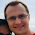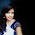Friday, July 31, 2009

Simple Newton's Method Fractal code in MATLAB

Due to popular request I've sharing some very simple Newton's Method Fractal code in MATLAB. It produces the following 800x800 image (in about 2.5 seconds on my 2.4Ghz Macbook Pro):

>> [niters,solutions] = matlab_fractal;
>> imagesc(niters)function [niters,solutions] = matlab_fractal
%Create Newton's Method Fractal Image
%Tomasz Malisiewcz (tomasz@cmu.edu)
%http://quantombone.blogspot.com/
NITER = 40;
threshold = .001;

[xs,ys] = meshgrid(linspace(-1,1,800), linspace(-1,1,800));
solutions = xs(:) + i*ys(:);
select = 1:numel(xs);
niters = NITER*ones(numel(xs), 1);

for iteration = 1:NITER
oldi = solutions(select);

%in newton's method we have z_{i+1} = z_i - f(z_i) / f'(z_i)
solutions(select) = oldi - f(oldi) ./ fprime(oldi);

%check for convergence or NaN (division by zero)
differ = (oldi - solutions(select));
converged = abs(differ) < threshold;
problematic = isnan(differ);

niters(select(converged)) = iteration;
niters(select(problematic)) = NITER+1;
select(converged | problematic) = [];
end

niters = reshape(niters,size(xs));
solutions = reshape(solutions,size(xs));

function res = f(x)
res = (x.^2).*x - 1;

function res = fprime(x)
res = 3*x.^2;

1.Just so you know: This code runs in Octave as well.

2.I imagined that it would run in Octave too but never tried. Thanks for trying out!

The reason why it runs fairly fast is that the Newton's Method iterations are vectorized over the pixels in the image. The only loop is over iterations. My additional speedup is to remove pixels from consideration once they have converged.

What's happening is that a complex number gets set for every pixel in the image and Newton's Method is ran to solve for the roots of the equation z^3-1=0 with the pixel's complex number as the initialization.

This image is then created by showing for every pixel how many iterations it took to converge. The other quantity of interest is which root was found; z^3-1=0 has three roots, one real and two complex. Showing the roots is a bit harder and to keep the code short and readable I used the iterations and Matlab's imagesc jet colorscheme.

3.hello sir, can you give me the MATLAB code for finding the complex (imaginary roots ) of a function? e.g --- x^2+25=0.

4.You can easily adapt the above code to do that for you via Newton's method. Just define f(x) and fprime(x) which is the derivative of f(x). I can't help you with the details -- it is not difficult.

5.If you type "imagesc(niters)" you will generate the picture

6.Hi. What is the licence of your code ?

7.8.